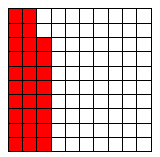### Home > MC1 > Chapter 4 > Lesson 4.3.3 > Problem4-108

4-108.

Use the Lesson 4.3.2B Resource Page to shade in the amounts represented by the following descriptions, and then write them in the stated form. .

1. Shade $7$-hundredths, and write the portion as a percent.

$7$-hundredths means you should shade $7$ boxes out of one hundred. Remember to write it as a percent.
For help, refer to the Math Notes box from Lesson 4.3.2.

1. Shade $7$-tenths, and write the portion as a fraction.

$7$ tenths is how many hundredths?

1. Shade $28$-hundredths, and write the portion as a fraction.

The correct amount has been shaded for you. Remember to write the portion as a fraction.1. Shade $31.5\%$, and write the portion as a decimal.

The decimal is $0.315$. Remember to shade $31.5\%$.

Use the eTool below to model each part.
Click the link at right for the full version of the eTool: 4-108 HW eTool